# How to Read and Understand Stem and Leaf Plots

How to Read and Understand Stem and Leaf PlotsEach number is separated into a stem and a leaf.

Write the last digit of each number in the leaf and the other digits are written in the stem.• A stem and leaf plot is a table in which numbers are arranged into a ‘stem’ and a ‘leaf’.
• Write the last digit of each number in the leaf.
• All digits in front of the last digit are written in the ‘stem’.
• The numbers must be written from smallest to largest.
• Numbers with the same front digits share a ‘stem’.
• For example, 10| 1 1 2 3 represents the numbers 101, 101, 102 and 103.
• 11| 4 5 represents the numbers 114 and 115.
• 12| 0 2 5 represents the numbers 120, 122 and 125.
• 13| 1 represents the number 131.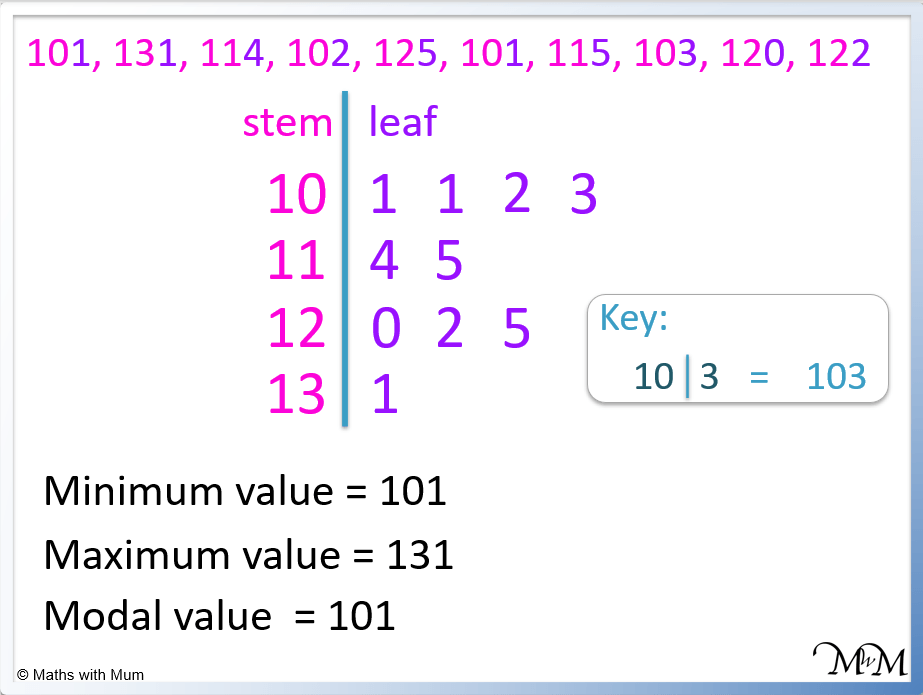• The numbers in a stem and leaf plot are always written from smallest to largest.
• The minimum is the first number in the plot, which is 101.
• The maximum is the last number in the plot, which is 131.
• The modal value is the number that comes up the most, which is 101.

Each number is represented by just one digit, written in the leaf part of the plot.

The numbers are listed from smallest to largest.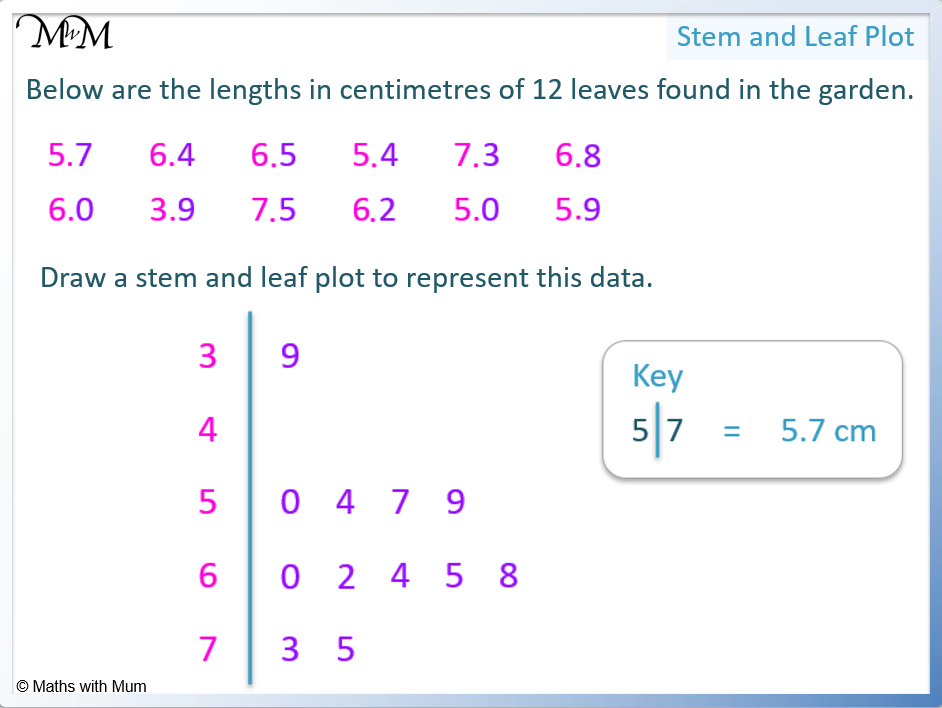• In a stem and leaf plot only the final digit is written in the leaf.
• In this example, the digit after the decimal point is written in the leaf and the digit before the decimal point is the stem.
• 3| 9 represents 3.9.
• The stem of 4| has no numbers in its leaf. This means that there are no numbers that start with a whole number of 4.
• 5| 0 4 7 9 represents the numbers 5.0, 5.4, 4.7 and 5.9.
• 6| 0 2 4 5 8 represents the numbers 6.0, 6.2, 6.4, 6.5 and 6.8.
• 7| 3 5 represents the numbers 7.3 and 7.5.Supporting Lessons

#### Stem and Leaf Plots: Video Lesson# Stem and Leaf Plots

## What is a Stem and Leaf Plot?

A stem and leaf plot is a table in which numbers are represented by one digit, listed in order. Each number is written with its first digits in the ‘stem’ and its final digit listed in the ‘leaf’. A stem and leaf plot is used to organise data visually so that the distribution, skew, outliers and mode can easily be seen.

The numbers in a stem and leaf diagram must be listed in order.A stem and leaf plot is read by combining the digits in the stem with the digit in the leaf.

Stem Leaf Numbers represented
10 1 1 2 3 101, 101, 102, 103
11 4 5 114, 115
12 0 2 5 120, 122, 125
13 1 131

Stem and leaf plots are used to display numerical data written as a list.

A stem and leaf plot displays all data values and this allows the mode to be easily read.When making a stem and leaf diagram, a key must always be included. A key is made by writing out one example from the diagram, including units. Keys are particularly useful if the numbers being represented contain many digits, units or are made from decimal numbers.

## How to Make a Stem and Leaf Plot

To make a stem and leaf plot:

1. Identify the minimum and maximum values in the data.
2. Create the stem by listing the first digits of the numbers.
3. Draw a line between the stem and the leaves.
4. Represent the numbers from smallest to largest by writing the final digit of each number in the correct leaf.
5. Create a key by writing an example to explain the diagram.

For example, make a stem and leaf plot to represent the following data showing the ages of people who at a park.

15, 1, 28, 7, 5, 33, 41, 4, 10, 35, 24, 2, 32, 6, 11, 4, 12, 5, 37, 30.

Step 1. Identify the minimum and maximum values in the data.

The minimum value is 1 and the maximum value is 41.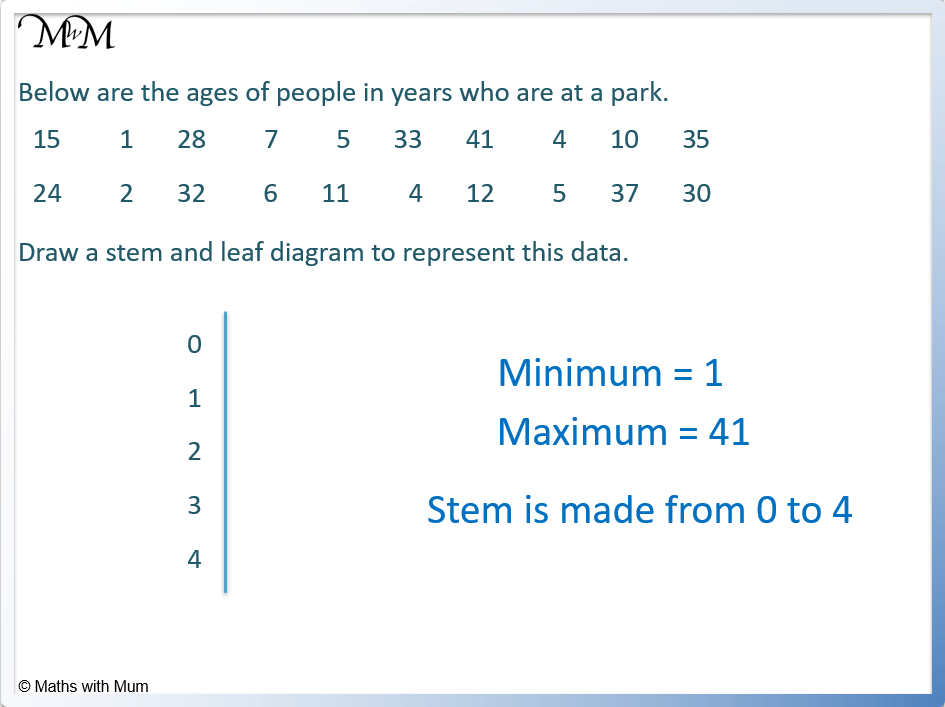Step 2. Create the stem by listing the first digits of the numbers.

The stem is made from all of the digits in the numbers except for the final digit.

In this example, the stem will be the tens place value column.

In the minimum value of 1, there are 0 tens.

In the maximum value of 41, there are 4 tens.

Therefore the stem is a list of numbers from 0 to 4.

The stem is made of 0, 1, 2, 3 and 4.

Step 3. Draw a line between the stem and the leaves.Step 4. Represent the numbers from smallest to largest by writing the final digit of each number in the correct leaf.

We start with the numbers with zero tens. We list them from smallest to largest.

The first number with zero tens is 1. We place it in the leaf alongside the 0 in the stem.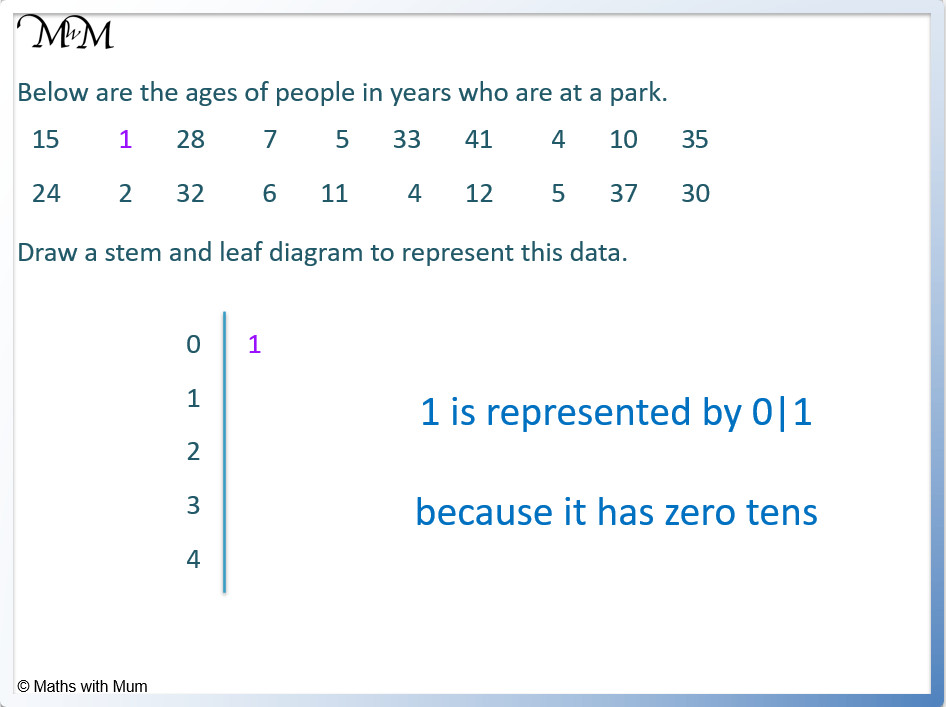Now we list the other numbers with zero tens.

The numbers are 2, 4, 4, 5, 5, 6 and 7. They are listed in order in line with the 0 in the stem.

If any numbers are repeated, they are still listed multiple times.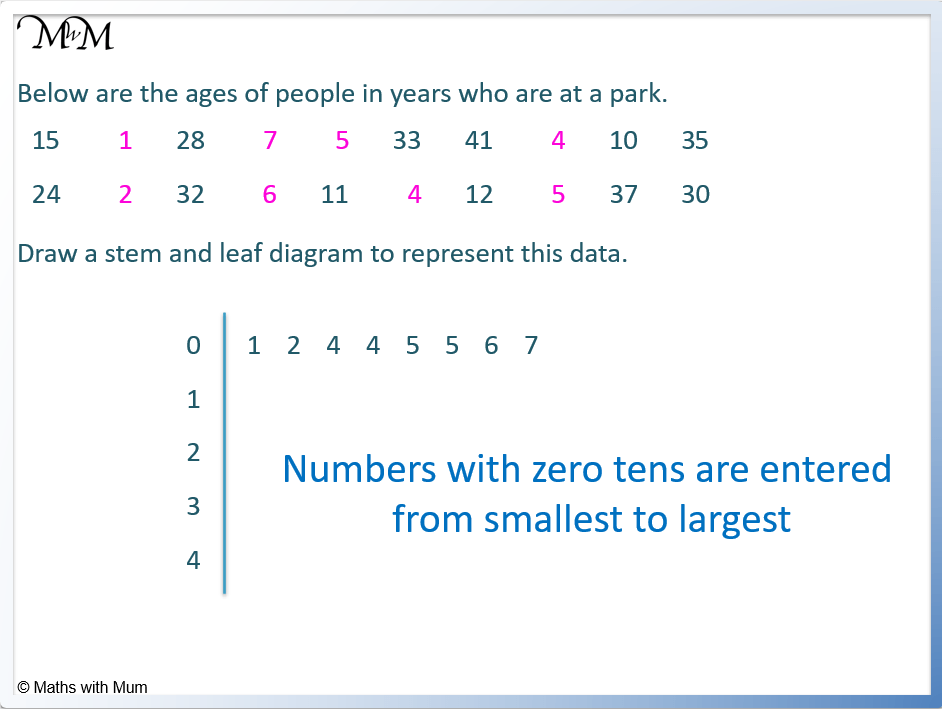Now we list the numbers with a 1 in the tens column.

We have 10, 11, 12 and 15.

We list the final digits of these numbers in line with the 1 in the stem.

10, 11, 12 and 15 are listed as 1| 0 1 2 5.

We only use the 1 in the tens column once in the stem of the diagram. The numbers are listed by their final digit only.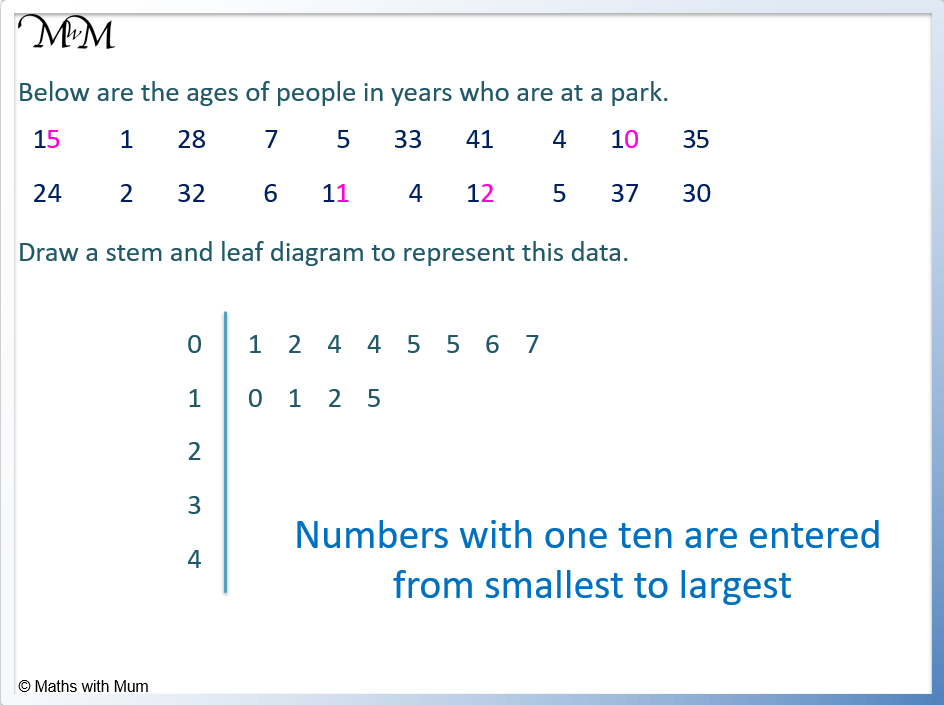Now we list the numbers with a 2 in the tens column.

24 and 28 are listed as 2| 4 8.Now we list the numbers with a 3 in the tens column.

30, 32, 33, 35 and 37 are represented as 3| 0 2 3 5 7.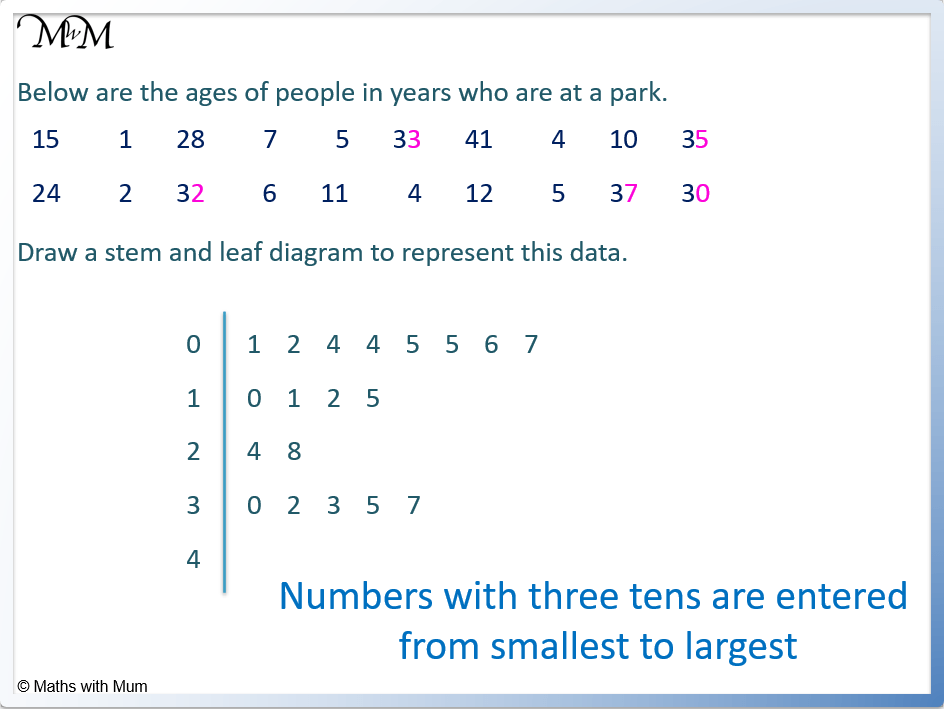Now we list the numbers with a 4 in the tens column.

We just have 41 which is listed as 4|1.Step 5. Create a key by writing an example to explain the diagram.

To make a key on a stem and leaf plot, write any number from the diagram and explain how it is read. For example 1|5 = 15.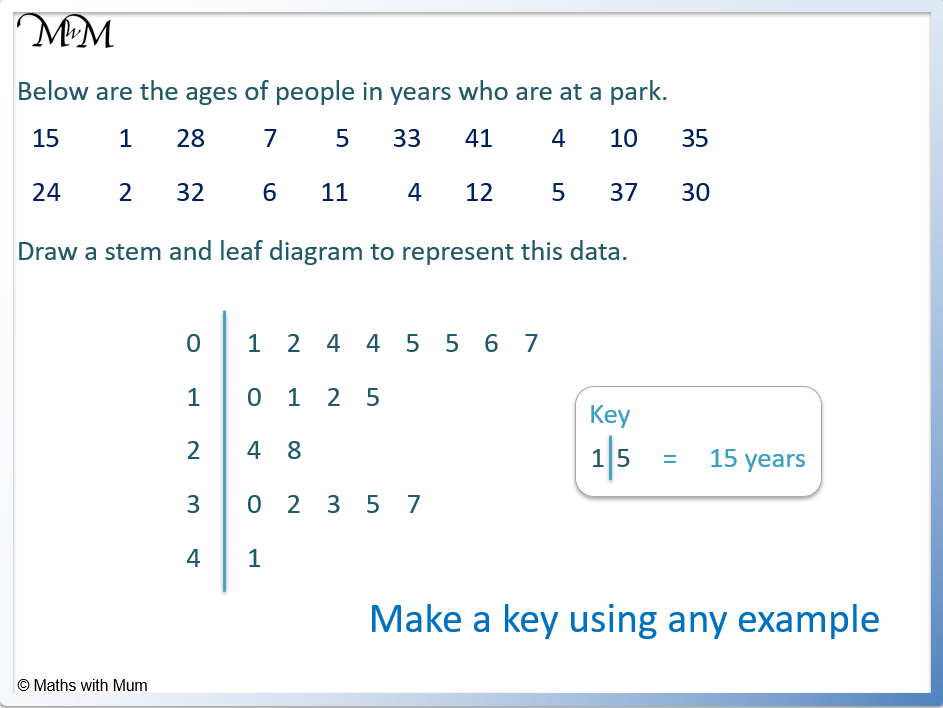## Interpreting a Stem and Leaf Plot

On a stem and leaf plot the stem is to the left of the vertical line and the leaf is to the right. Combine the digits in the stem with each digit in the leaf to read each number.#### How to Find the Range on a Stem and Leaf Plot

The range is the maximum value subtract the minimum value. On a stem and leaf plot, the minimum is the first value and the maximum is the last value. For example if the maximum value is 41 and the minimum value is 1, the range is 41 – 1 which equals 40.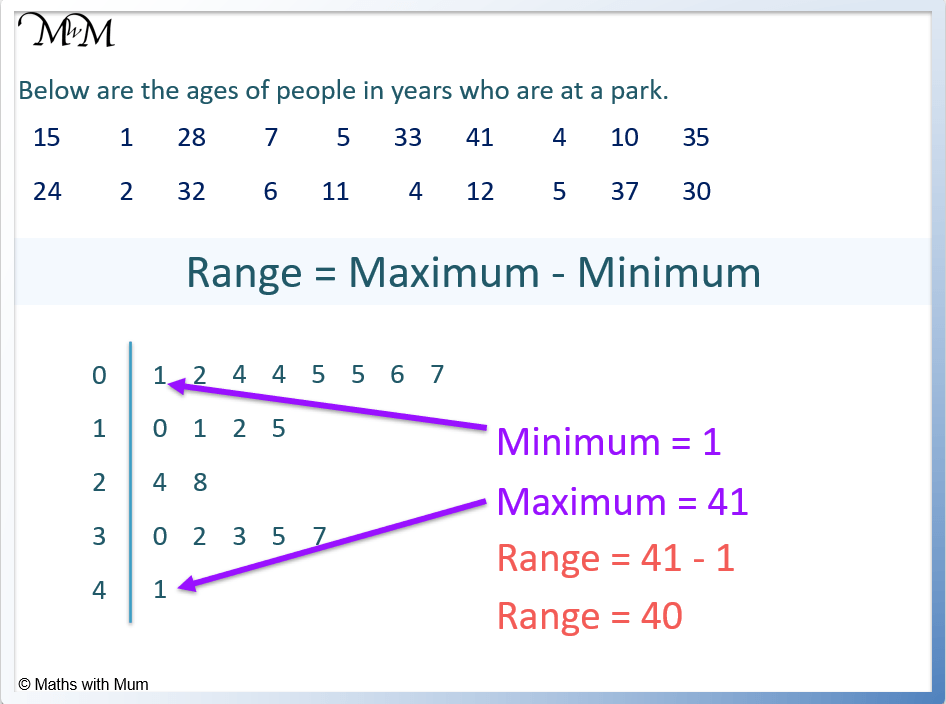Here is another example of finding the range on a stem and leaf diagram.

The range= maximum – minimum.

The maximum is $55 and the minimum is$12.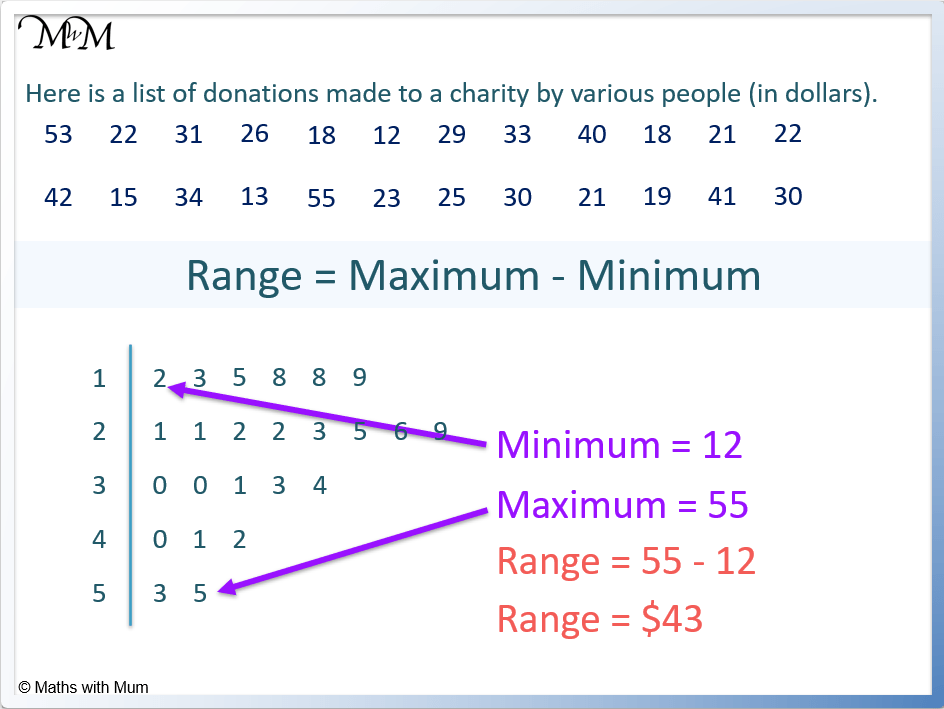The range = 55 – 12 = 43.

The range equals $43. #### How to Find the Median on a Stem and Leaf Plot The median is the middle value in a set of ordered data. To find the median on a stem and leaf diagram, cross off the same amount of values at the start and end of the diagram until one value is left. If two values are left, the median is found directly between them. This can be calculated by adding the two values and dividing by two. For example, on the following stem and leaf diagram there are 19 values. We cross off 9 values at the start and 9 values of the end. This leaves the value of 22 in the middle. The median is 22.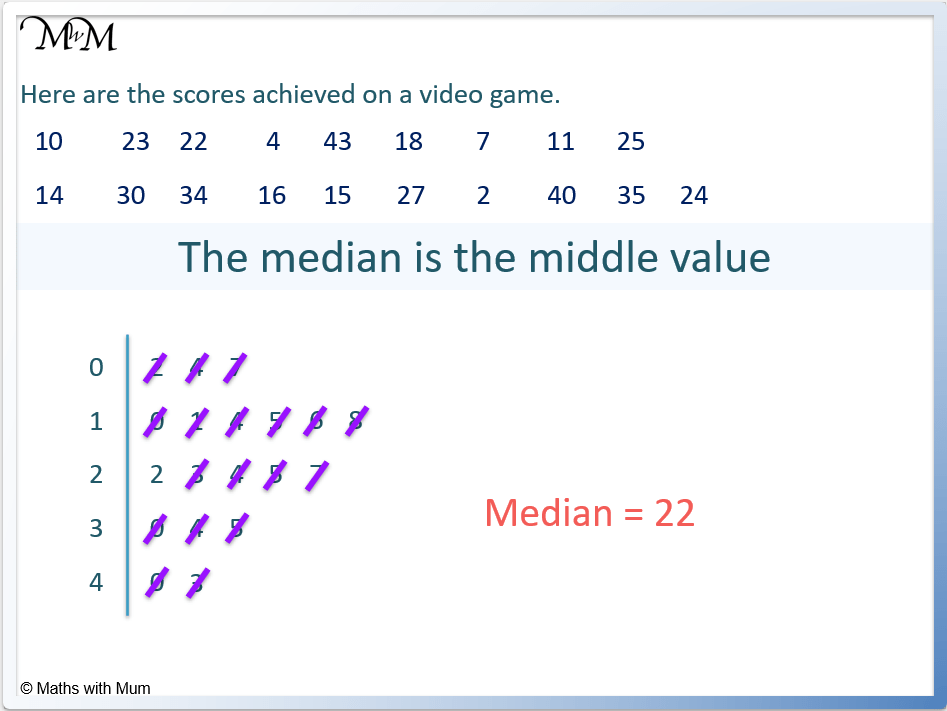The median can be found on a stem and leaf plot at the (n+1)/2 position, where n is the number of data values. In the example above, there are 19 values and so, n = 19. The median is found at the (19+1)/2 position. This means that the median is found at the 10th position. Counting 10 numbers in, we get to the median of 22. Here is another example of finding the median from a stem and leaf plot with an even number of data values. There are 24 numbers in the stem and leaf diagram. We can cross off 11 numbers at the start and 11 numbers at the end to leave 25 and 26 in the middle. The median is found directly between 25 and 26 at 25.5. The median donation is$25.50.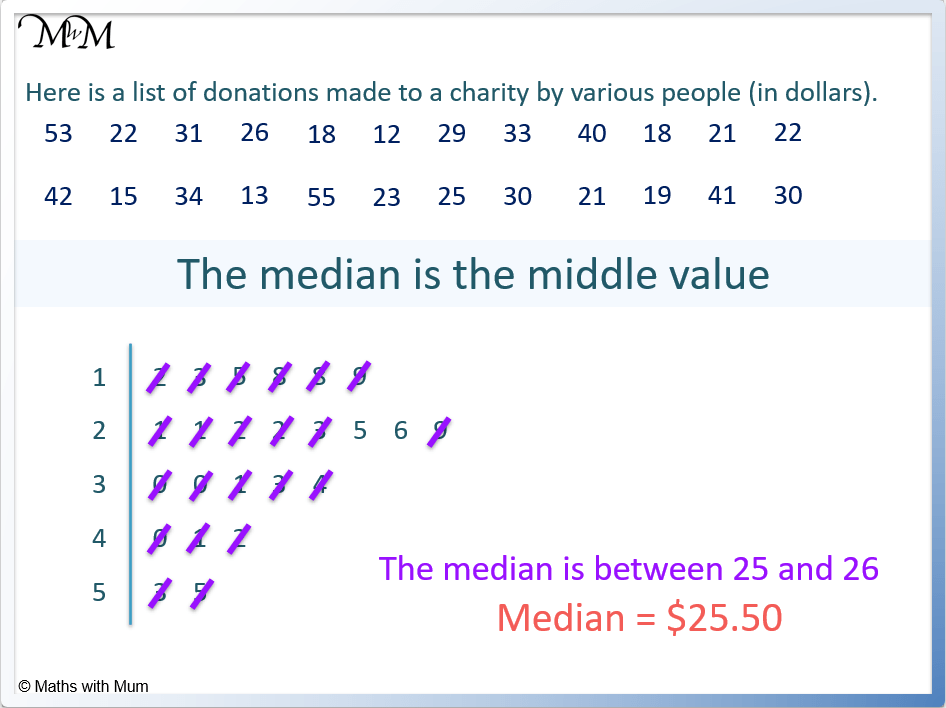#### How to Find Quartiles on a Stem and Leaf Plot

• The lower quartile is found at the 1/4 (n+1)th term on a stem and leaf plot.
• The upper quartile is found at the 3/4 (n+1)th term.
• The interquartile range is equal to the upper quartile subtract the lower quartile.

Here is an example of a stem and leaf plot with 19 data values. n = 19.

The lower quartile is found at the 1/4 (n+1)th term. We substitute n = 19 into the formula.

1/4 (19+1) = 5 and so, the lower quartile is found at the 5th term.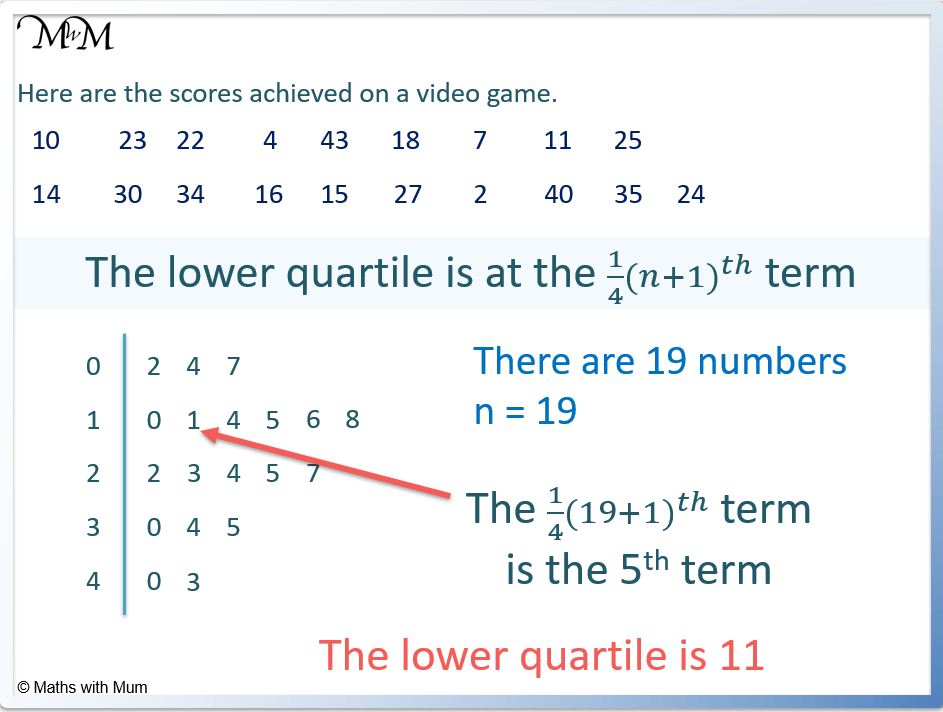The 5th term is 11. The lower quartile is 11.

The upper quartile is found at the 3/4 (n+1)th term. We substitute n = 19 into the formula.

3/4 (19+1) = 15 and so, the lower quartile is found at the 15th term.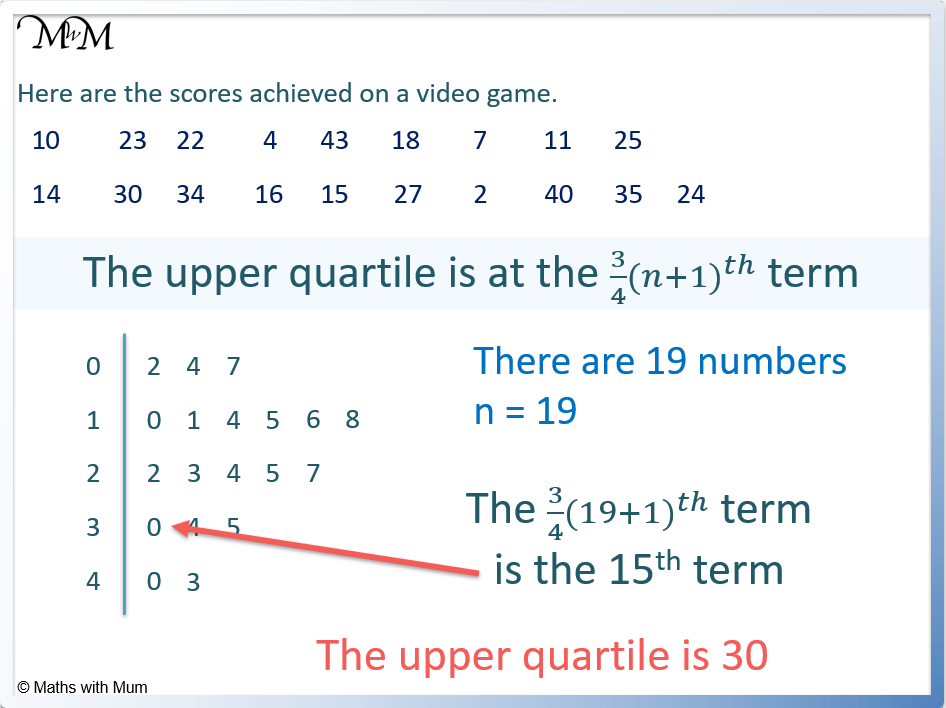The 15th term is 30. The upper quartile is 30.

The interquartile range is equal to the upper quartile – lower quartile.

IQR = 30 – 11.

IQR = 19.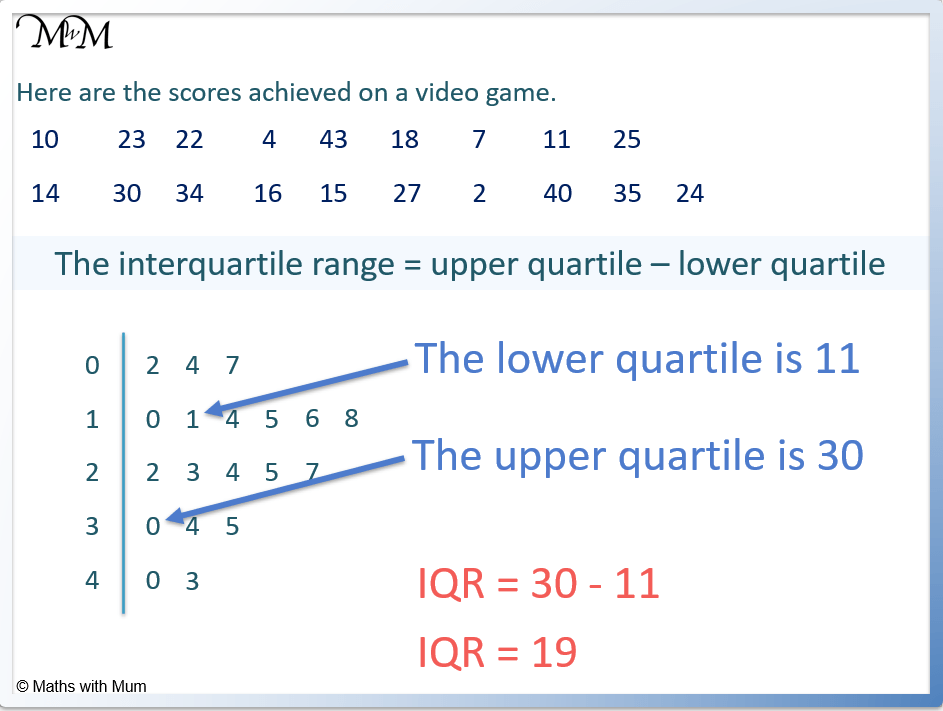#### Skewness of a Stem and Leaf Diagram

On a stem and leaf plot, data is skewed when the majority of the data is clustered at the start or end of the plot. If the majority of the data is found at the start of the plot, the data has right (or positive) skew. If the majority of the data is found at the end of the plot, the data has left (or negative skew). If amount of data is the same at each end, it is symmetrical.To find the skew of a stem and leaf plot, draw a line over the top of the data. Look at the shape of the line drawn. If it is clustered at the start of the data, it is right (or positively) skewed and if it is clustered at the end of the data, it is left (or negatively) skewed.

For example, what is the skew of the following stem and lead plot?We draw a line over the data to see its distribution.

There is a peak in the data at the start of the plot. Therefore the data is right (or positively) skewed.

Not all stem and leaf plots show skew. Below is an example of a stem and leaf diagram with no skew.## Back-to-Back Stem and Leaf Plots

A back-to-back stem and leaf plot is used to compare two sets of data. One set of data is shown in the leaves to the right of the stem, whilst the other set of data is shown in the leaves to the left of the stem. Both sets of data are arranged from smallest to largest, with the values getting larger as they move away from the stem.

For example, here is a back-to-back stem and leaf plot showing the test results of students in two different classes.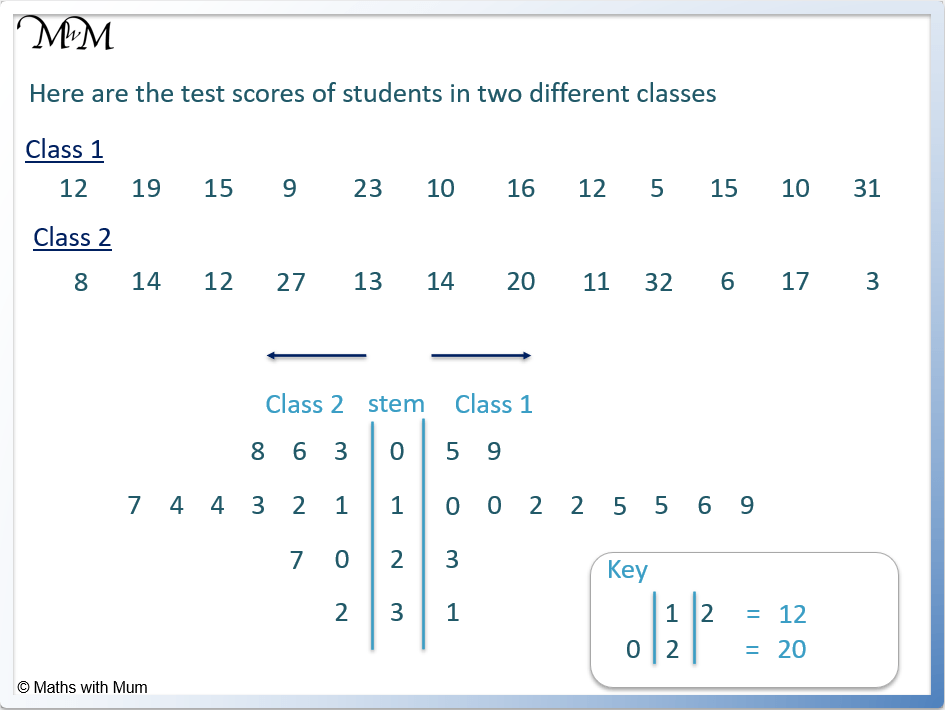Class 1 is shown on the right of the stem and class 2 is shown on the left of the stem.

The data is always written from smallest to largest as we move away from the stem. The data for class 1 gets larger as we move right and the data for class 2 gets larger as we move left.

The key helps to explain the data values on each side of the back-to-back stem and leaf plot.

For example, reading the data for class 1: |1|2 means 12.

Read the digits backwards on the left side of a back-to-back stem and leaf diagram. For example, 0|2| means 20.

Here is an example of a back-to-back stem and leaf plot with decimals.

The data for boys is written on the right of the stem, getting larger from left to right.

The data for girls is written on the left of the stem, getting larger from right to left.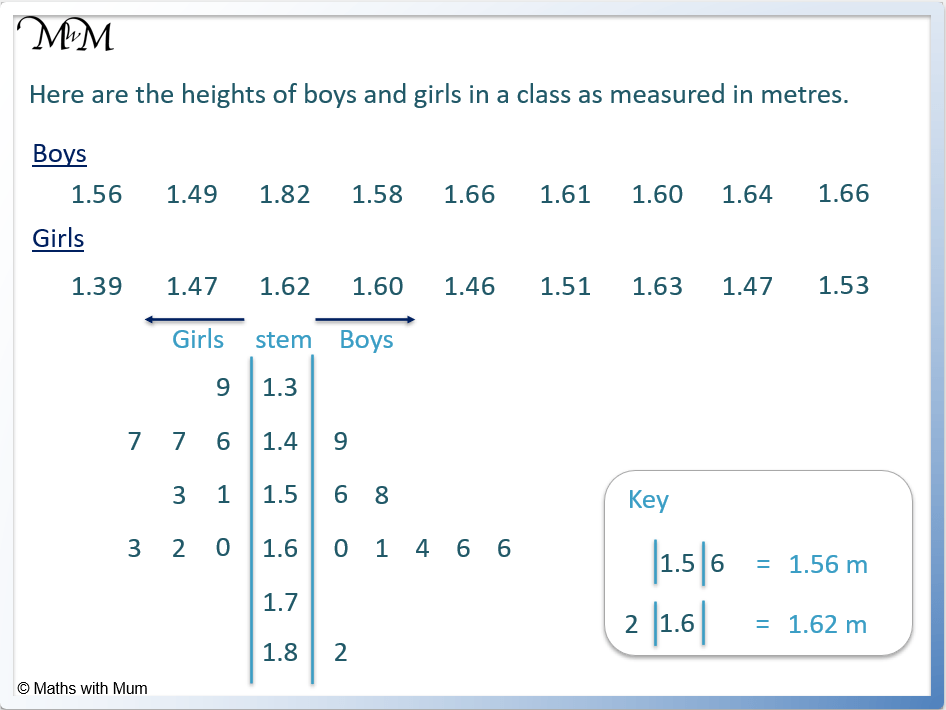For example, |1.5|6 means 1.56 m.

For example, 2|1.6| means 1.62 m. Again, we read backwards when reading the left leaves on a back-to-back stem and leaf plot.

#### Stem and Leaf Plot with Hundreds

To represent numbers in the hundreds on a stem and leaf plot, write the hundreds and tens digits in the stem and write the ones digit in the leaf.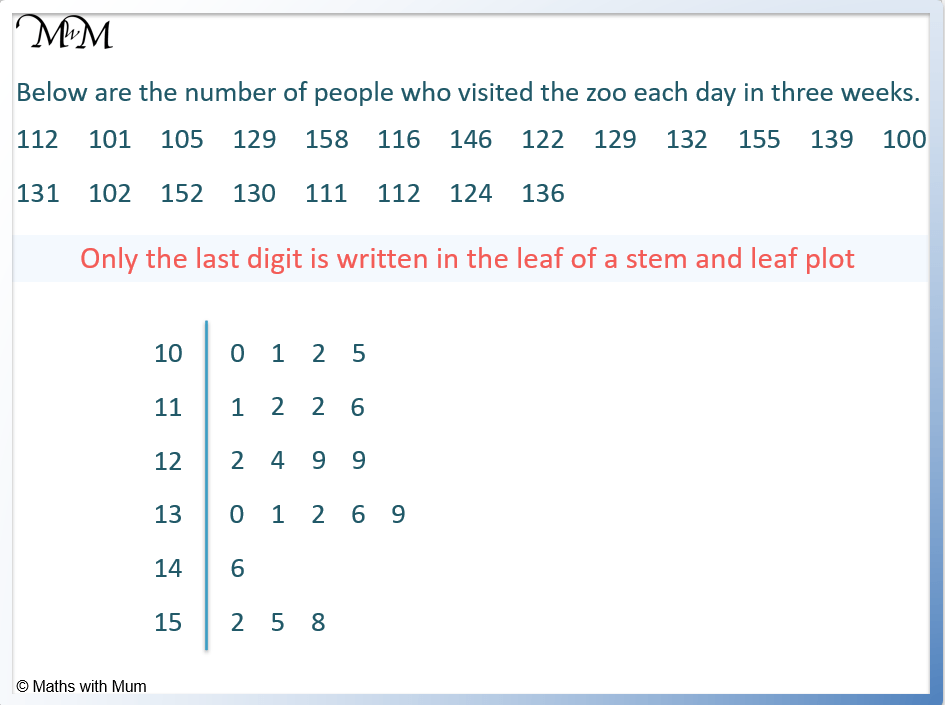For example, 12|4 means 124.

#### Stem and Leaf Plot with Thousands

To represent numbers with thousands on a stem and leaf plot, write the thousands, hundreds and tens digits in the stem and write the ones digit in the leaf.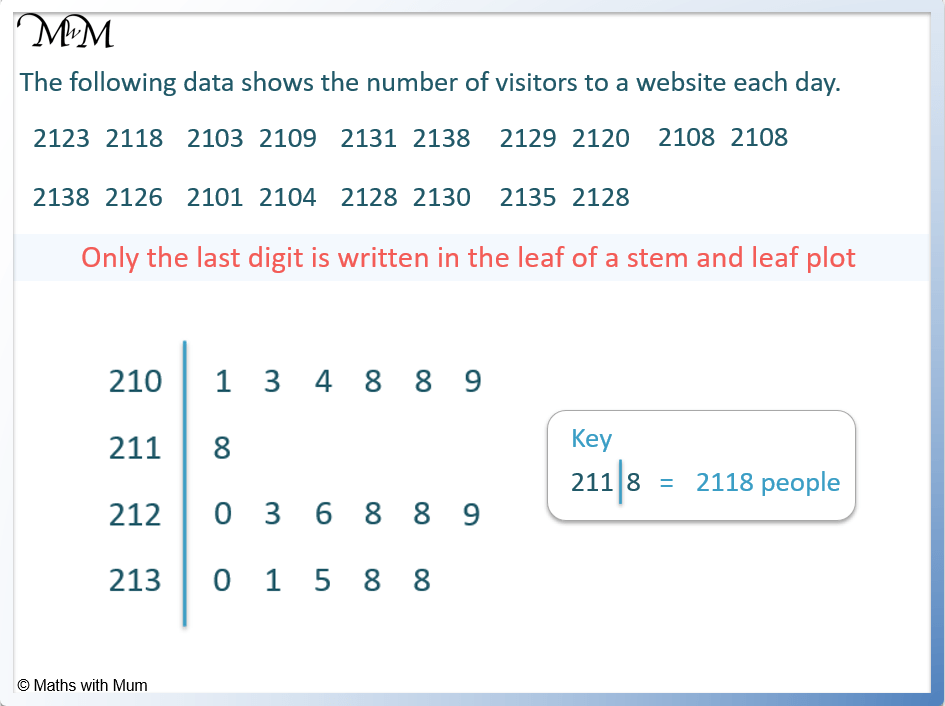For example, 211|8 means 2118.

## Stem and Leaf Plots with Decimals

To write decimal numbers in a stem and leaf plot, write the final decimal digit in the leaf. Write the preceding digits in the stem. The decimal point may also be included in the stem. However, if the numbers only have one decimal place, the decimal point is not included.

#### Stem and Leaf Plots with 1 Decimal Place

To write decimal numbers with 1 decimal place on a stem and leaf plot, write the whole number digits in the stem and the single decimal digit in the leaf.

For example, 5|7 means 5.7. The line between the stem and the leaf acts as the decimal point.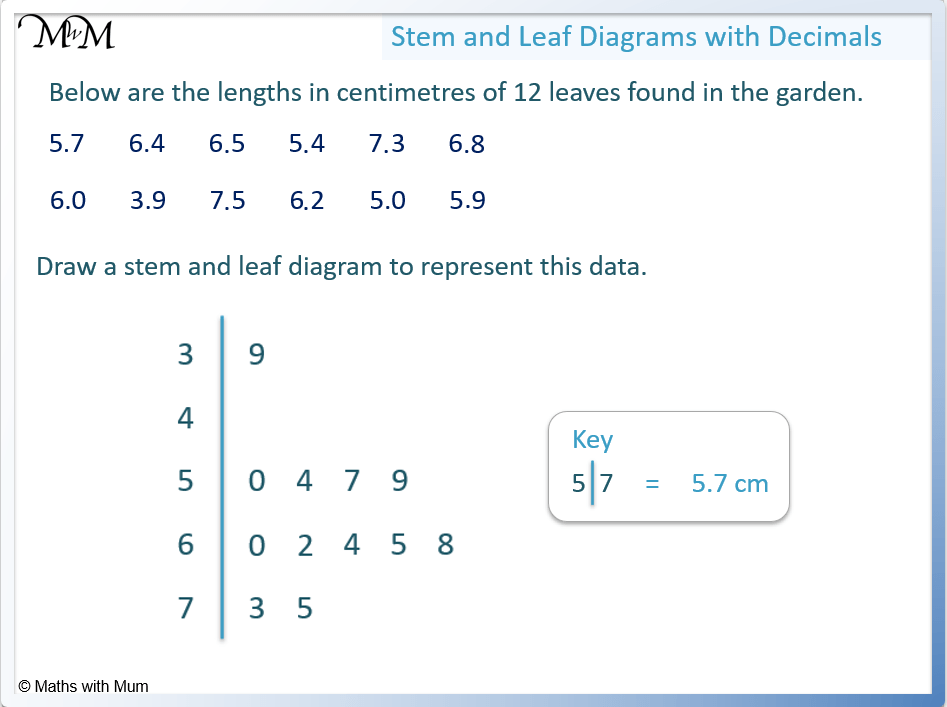#### Stem and Leaf Plots with Multiple Decimal Places

To represent a number with multiple decimal places on a stem and leaf plot, write the final digit of each number in the leaf part of the plot. Write all preceding digits including the decimal point in the stem.

For example, 1.5|6 means 1.56.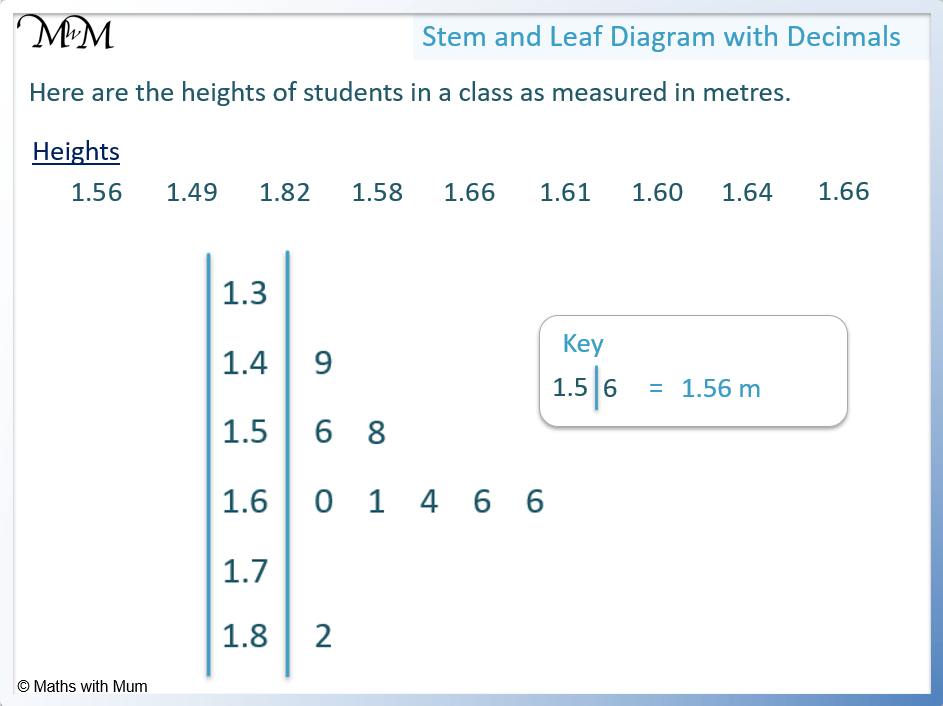Now try our lesson on How to Read Coordinates where we learn how to read coordinates on a graph.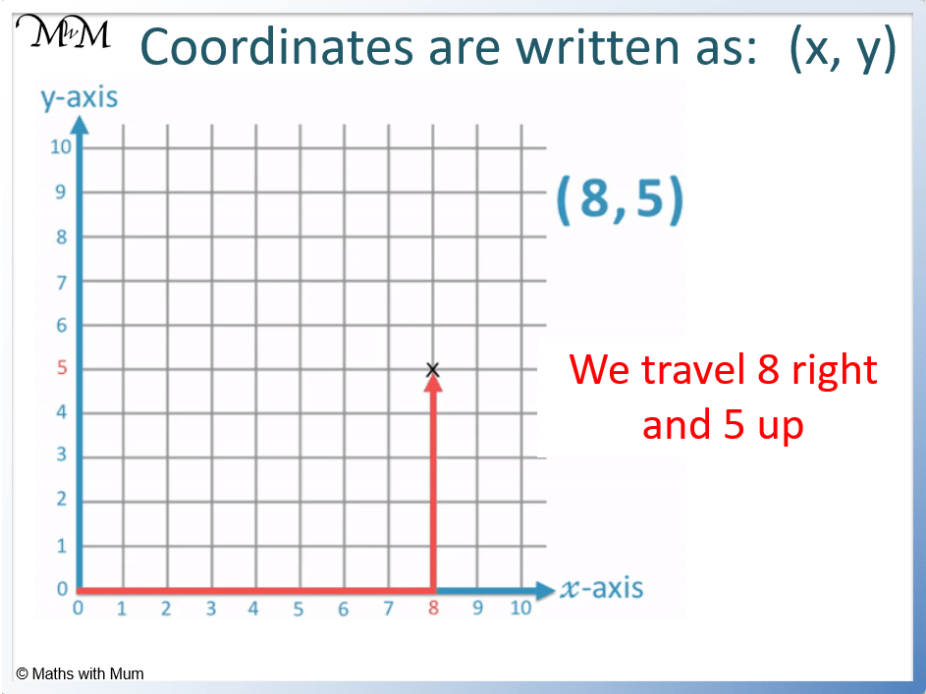error: Content is protected !!